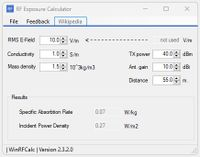# SAR (Specific Absorption Rate) calculator.The SAR (Specific Absorption Rate) is calculated from the level of the electric field (E-Field), the conductivity (Siemens per meter) of the biological material and the mass-density in kg/m3.

In case of a radiating antenna with known transmit power, antenna gain and distance to the target the resulting E-field can be calculated as an input to the SAR evaluation.

>100 RF Calculators
WinRFCalc, the best RF calculator toolbox for Windows